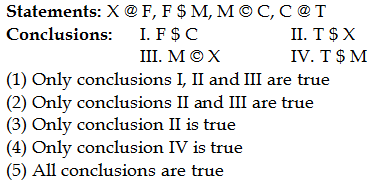## PREPARATORY QUESTIONS

### EQUAL-INEQUAL

#### PREPARATORY SET-30 (HARD)

Direction (Qs.1 to 5): In the given questions, the symbols @, \$, and Â©, % and # are used with the following meanings as illustrated below.

‘M \$ N’ means ‘M is neither smaller than nor equal to N’

‘M # N’ means ‘M is not smaller than N’

‘M @ N’ means ‘M is not greater than N’

‘M % N’ means ‘M is neither smaller than nor greater than N’

‘M © N’ means ‘M is neither greater than nor equal to N’

Now in each of the given questions assuming the given statements to be true, find which of the two conclusions I and II given below them is/are definitely true.

Question No : 1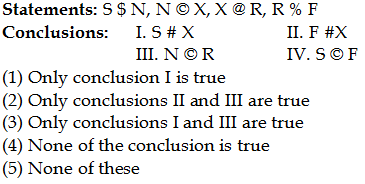Question No : 2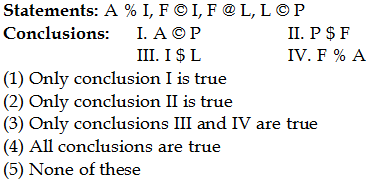Question No : 3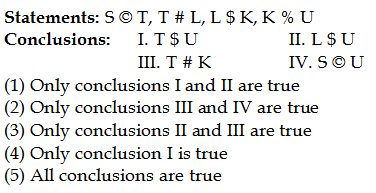Question No : 4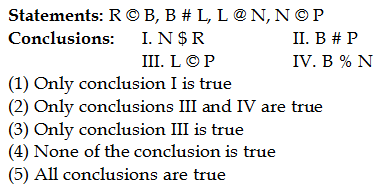Question No : 5